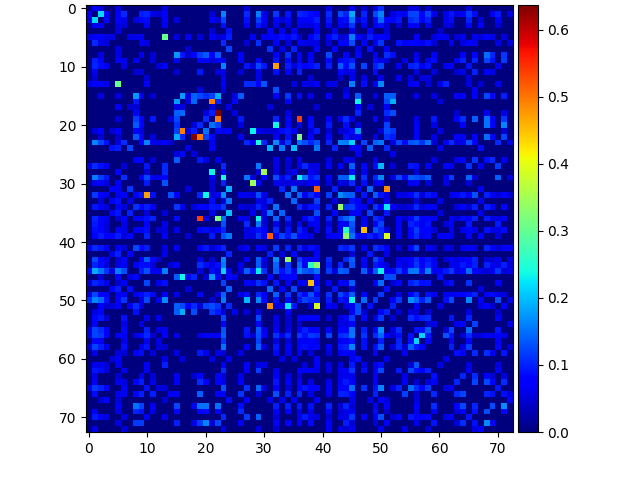# How can I plot in root/CERN a matrix from top to bottom like in python, i.e. the zero on the y-axis is at the top. thanks a lotFIGURA (1: This is the graph in python )

FIGURA(2:This is the graph in ROOT. I want the zero on the y-axis to be equal to the graph in python.)

There is no simple option in ROOT to do that. Nevertheless, this example may help you.

Hi @couet, thanks for replying. Your solution is interesting, but I’m afraid that this option is only to invert the axes, without affecting the chart, so, as I see things, now I would have to also implement an option to rotate the chart, additionally relocate the color palette, a big job.

May be something like that could help:

``````void ReverseXAxis (TH1 *h);
void ReverseYAxis (TH1 *h);
void DrawReverseBins (TH1 *h);

void reverseaxis()
{
TH2F *hpxpy  = new TH2F("hpxpy","py vs px",40,-4,4,40,-4,4);
Float_t px, py;
TRandom r;
for (Int_t i = 0; i < 25000; i++) {
r.Rannor(px,py);
hpxpy->Fill(px,py);
}
TCanvas *c1 = new TCanvas("c1");
hpxpy->Draw("colz");
DrawReverseBins(hpxpy);
ReverseXAxis(hpxpy);
ReverseYAxis(hpxpy);
}

void DrawReverseBins (TH1 *h)
{
TH2F *h2  = new TH2F(h->GetName(),h->GetTitle(),
h->GetNbinsX(),0,1,h->GetNbinsX(),0,1);

h2->GetXaxis()->SetLabelOffset(999);
h2->GetXaxis()->SetTickLength(0);
h2->GetYaxis()->SetLabelOffset(999);
h2->GetYaxis()->SetTickLength(0);

for (int i=1; i<=h->GetNbinsX(); i++) {
for (int j=1; j<=h->GetNbinsY(); j++) {
h2->SetBinContent(j,i,h->GetBinContent(i,j));
}
}

h2->Draw("colz");
}

void ReverseXAxis(TH1 *h)
{
TGaxis *newaxis = new TGaxis(gPad->GetUxmax(),
h->GetXaxis()->GetXmin(),
h->GetXaxis()->GetXmax(),
510,"-");
newaxis->SetLabelOffset(-0.03);
newaxis->Draw();
}

void ReverseYAxis(TH1 *h)
{